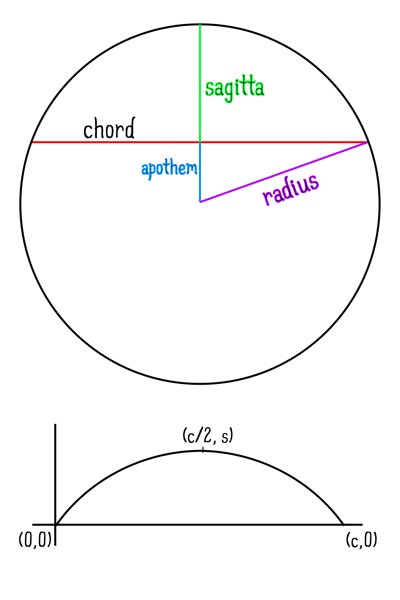# The Mathematics of Arches

Arches are part of the design feature set of our house — so is a mansard roof, but I am not as keen to replicate it — and as I add or replace various features on the house, I would like to add the same kind of flattened arch that features on facade of the house and in some of the cabinetry. For that, I need math. In particular, given the width of a given opening and how high I would like the arch to be, I need to be able to calculate the length of material of the resulting arch.

For those who missed this particular part of geometry, here are the parts involved:For the math, we need the following:

[code lang=text]
(x – x)^2 + (y – y)^2 = r^2
[/code]

Or:

[code lang=text]
x = c/2

y = (s – x^2/s) / 2

r^2 = x^2 + y^2

Y = y + sqrt(r^2 – (x – x)^2)
[/code]